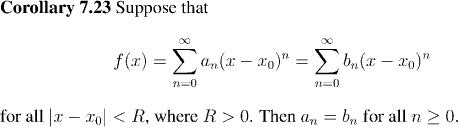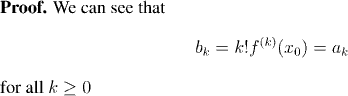Analysis WebNotes
arrow_backRemark:
We didn't need to assume that the two series had the same radius of convergence, as long as both of them had positive radius of convergence. By applying the argument above for the smaller of the two radii of convergence, you deduce that the coefficients of the two series are the same (and so the radii of convergence had to be the same). On the other hand, we do need to assume that the two power series are centered on the same point x0.